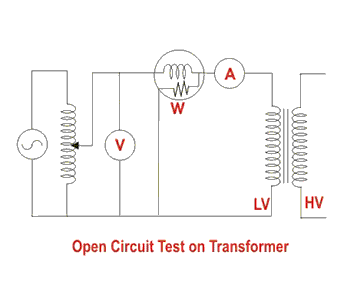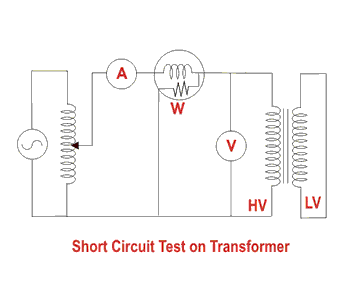# OC & SC Test of Transformer

Transformer is static machine which pass the electrical energy from one place to another place without change the frequency. Generally Transformer having two types of test,

1. Open circuit test
2. Short circuit test

### Open circuit (OC) test

###### The shunt branch parameters can be determined by performing this test. Since, the core loss and the magnetizing current depend on applied voltage, this test is performed by applying the rated voltage to one of the windings keeping the other winding open (generally HV winding is kept open and rated voltage is applied to LV winding). The circuit diagram to conduct this test. Since, the secondary terminals are open (no load is connected across the secondary), current drawn from the source is called as no load current.###### On no-load, the approximate equivalent circuit.  can be further reduced. Under no-load condition the power input to the transformer is equal to the sum of losses in the primary winding resistance R1,  and core loss. Since, no load current is very small, the loss in winding resistance is neglected. Hence, on no load the power drawn from the source is dissipated as heat in the core. If Io and Pi are the current and input power drawn by the transformer at rated voltage V1 respectively, then

Iμ = I0sinΦ0   and    Iw = I0cosΦ0.
cosΦ0 (no load power factor) = W / (V1I0). … (W = wattmeter reading)
From this, shunt parameters of equivalent circuit parameters of equivalent circuit of transformer (X0 and R0) can be calculated as
X0 = V1/Iμ  and  R0 = V1/Iw.

(These values are referring to LV side of the transformer.)
Hence, it is seen that open circuit test gives core losses of transformer and shunt parameters of the equivalent circuit.

### Short circuit (SC) test

###### Suppose the input voltage is reduced to a small fraction of rated value and secondary terminals are short-circuited. A current will circulate in the secondary winding. Since a small fraction of rated voltage is applied to the primary winding, the °ux in the core and hence the core loss is very small. Hence, the power input on short circuit is dissipated as heat in the winding. The circuit diagram to conduct this test . In this test, the LV terminals of the transformer are short circuited. The primary voltage is gradually applied till the rated current °ows in the winding. Since, the applied voltage is very small ( may be of the order of 5¡8%), the magnetizing branch can now be eliminated from the equivalent circuit. The modi¯ed equivalent circuit. If Vsc is the applied voltage to circulate the rated current (I02) on short circuit, and Pc is the power input to the transformer.Therefore, W = Isc2Req……. (where Req is the equivalent resistance of transformer)
Zeq = Vsc/Isc.
Therefore, equivalent reactance of transformer can be calculated from the formula  Zeq2 = Req2 + Xeq2.